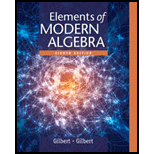# 25 . Let a and b be a real number, and let n be integers with 0 ≤ r ≤ n . The binomial theorem states that ( a + b ) n = ( n 0 ) a n + ( n 1 ) a n − 1 b + ( n 2 ) a n − 2 b 2 + ... + ( n r ) a n − r b r + ....... + ( n n − 2 ) a 2 b n − 2 + ( n n − 1 ) a b n − 1 + ( n n ) b n = ∑ r = 0 n ( n r ) a n − r b r Where the binomial coefficients ( n r ) are defined by ( n r ) = n ! ( n − r ) ! r ! , With r ! = r ( r − 1 ) ......... ( 2 ) ( 1 ) for r ≥ 1 and 0 ! = 1 . Prove that the binomial coefficients satisfy the equation ( n r − 1 ) + ( n r ) = ( n + 1 r ) for 1 ≤ r ≤ n This equation generates all the “"interior “"entries (printed in bold) of Pascal’'s triangle. 1 1 1 1 2 1 1 3 3 1 1 4 6 4 1 1 5 1 0 1 0 5 1 ⋰ ⋮ ⋱### Elements Of Modern Algebra

8th Edition
Gilbert + 2 others
Publisher: Cengage Learning,
ISBN: 9781285463230### Elements Of Modern Algebra

8th Edition
Gilbert + 2 others
Publisher: Cengage Learning,
ISBN: 9781285463230

#### Solutions

Chapter
Section
Chapter 2.2, Problem 25E
Textbook Problem

## Expert Solution

### Want to see the full answer?

Check out a sample textbook solution.See solution

### Want to see this answer and more?

Experts are waiting 24/7 to provide step-by-step solutions in as fast as 30 minutes!*

See Solution

*Response times vary by subject and question complexity. Median response time is 34 minutes and may be longer for new subjects.